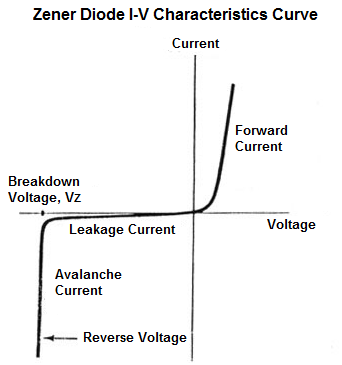﻿ What is a Zener Diode?

# What is a Zener Diode?A Zener Diode is a special type of diode that is used most extensively as a voltage regulator; this is because once the reverse voltage supplied to a zener diode reaches its breakdown voltage, referred to as VZ, the voltage across the diode remains very constant at this voltage even if the current through the diode continues to increase or vary.

For this reason of steady voltage after its breakdown voltage is this diode able to function as a voltage-regulating device.

Other diodes are not optimized to function with constant voltage after the reverse voltage has exceeded their breakdown voltages and will not display this characteristic that a zener diode contains.

Below is the voltage-current characteristics curve of a zener diode:You can see in the above curve the how steady and constant the voltage across the zener diode is after it reaches the breakdown voltage, despite large changes in current.

This makes the zener diode very useful in circuits where steady voltages need to be supplied.

The zener voltage of zener diodes comes in a range of values. You can find them in 3.3V-12V easily in widespread use.

The circuit below has a zener voltage of 5.1V.Therefore, the 12-volt power supply drops 5.1V and the voltage across the zener remains constant at this voltage. This 5.1 zener voltage is then placed in parallel to a load device, which it powers. This is a voltage regulator circuit.

The zener voltage of the diode can be any voltage, as is needed to be constant and power a circuit.

Related Resources